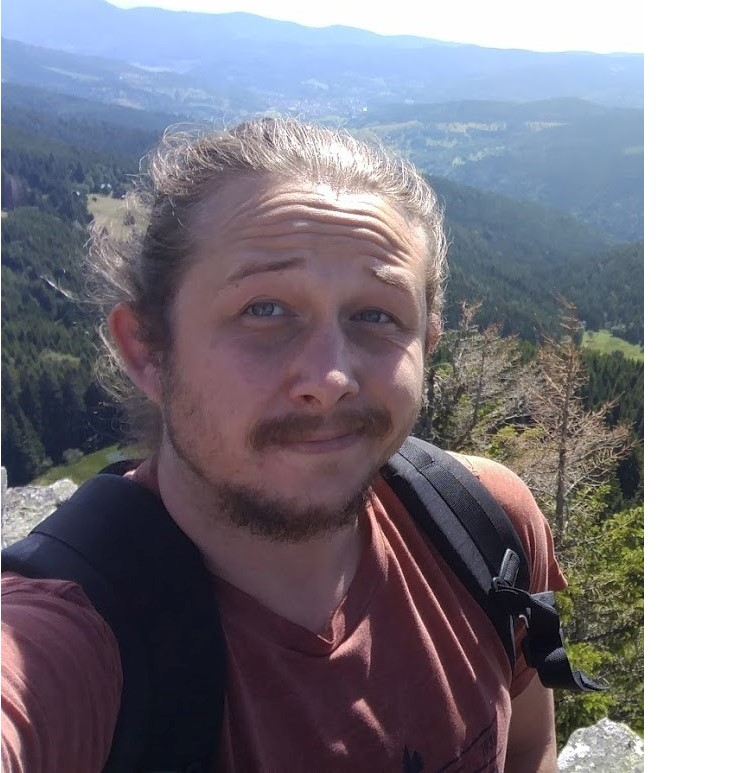# PhD Defence: Igor Khmelnitsky

Verification of Infinite-State Systems and Machine Learning
by Igor Khmelnitsky
Thursday 27 January 2022 at 2pm
Room 1Z25, ENS Paris-Saclay and onlineAbstract: This work consists of three parts. The first one is devoted to the verification of Petri nets, the second one to the verification of recursive Petri nets which extend Petri nets, and the final one aims at combining active learning and verification.

A Petri net can be analyzed by computing and studying its Clover, that is the canonical representation of the downward over approximation of its reachability set. Using the Karp-Miller algorithm one can compute the Clover, but this algorithm is very inefficient and moreover, its original proof of correctness is not satisfying. Many variations of the original Karp-Miller algorithm computing the clover exist, but some are incomplete, others introduced an unknown supplementary memory size (possibly Ackermannian) and proofs are often heavy. Our first contribution is the design of a complete algorithm in such a way that we can theoretically bound the additional memory requirements. The key idea of this algorithm is the introduction of a new concept, called acceleration. More precisely using accelerations, we were able:

1. to simplify the proof of correctness of the Karp-Miller algorithm
2. to present the first simple modification of the original but incomplete Minimal Coverability Tree algorithm
3. to prove that the supplementary memory needed by our algorithm is elementary (2-EXPSPACE)
4. to implement a prototype MinCov, showing experimentally that it is the most efficient one compared to other tools computing the Clover.

In the early two-thousands, Recursive Petri nets (RPN) have been introduced in order to model distributed planning of multi-agent systems for which counters and recursivity were necessary. Although RPN strictly extend Petri nets and context-free grammars, most of the usual problems (reachability, termination, etc.) were shown to be decidable. For almost all other models extending Petri nets and context-free grammars, the complexity of coverability and termination are unknown or strictly larger than EXPSPACE. In contrast, we establish here that for RPN, the coverability, termination, boundedness and finiteness problems are EXPSPACE-complete as for Petri net. While having a great expressive power, RPN suffer several modeling limitations. We introduce Dynamic Recursive Petri nets (DRPN) which address these issues extending the expressiveness of RPN. This model generalizes almost all previous known models, which extend the Petri net and keep the coverability problem decidable. Thus we establish that the coverability problem is decidable for DRPN.

For active learning and formal methods, our work focuses on Angluin’s L* algorithm. Angluin’s algorithm learns the minimal deterministic finite automaton (DFA) of a regular language using membership and equivalence queries. Its probabilistic approximately correct (PAC) version substitutes an equivalence query by a set of random membership queries. Thus, it can be applied to any kind of device and may be viewed as synthesizing an automaton from observations of the device. We are interested in how the PAC version behaves for devices which are obtained from a DFA by introducing some noise. More precisely, we study whether the algorithm reduces the noise, producing a DFA closer to the original one than the noisy device. We found that the reduction of the noise strongly depends on the type of noise and its amount. Moreover, we use this algorithm to develop a property-directed approach for verification of recurrent neural networks (RNNs). It learns a DFA as a surrogate model from a given RNN, which is then analyzed using model checking as a verification technique. We show that this not only allows us to discover small counterexamples fast, but also to generalize them by pumping towards faulty flows, hinting at the underlying error in the RNN.

### Jury:

• Javier ESPARZA, Professor, Technische Universität München - Reviewer
• Ranko LAZIC, Professor, University of Warwick - Reviewer
• Patricia BOUYER-DECITRE, Research Director, CNRS, ENS Paris-Saclay, Université Paris-Saclay - Examiner
• Dana FISMAN, Associate Professor, Ben-Gurion University - Examiner
• Laure PETRUCCI, Professor, Université Sorbonne Paris Nord - Examiner
• Pierre-Alain REYNIER, Professor, Aix Marseille Université - Examiner
• Alain FINKEL, Professor, Université Paris-Saclay - PhD supervisor